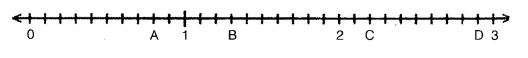# Write the decimal number represented by the points A, B, C, D on the given number line

Write the decimal number represented by the points A, B, C, D on the given number line